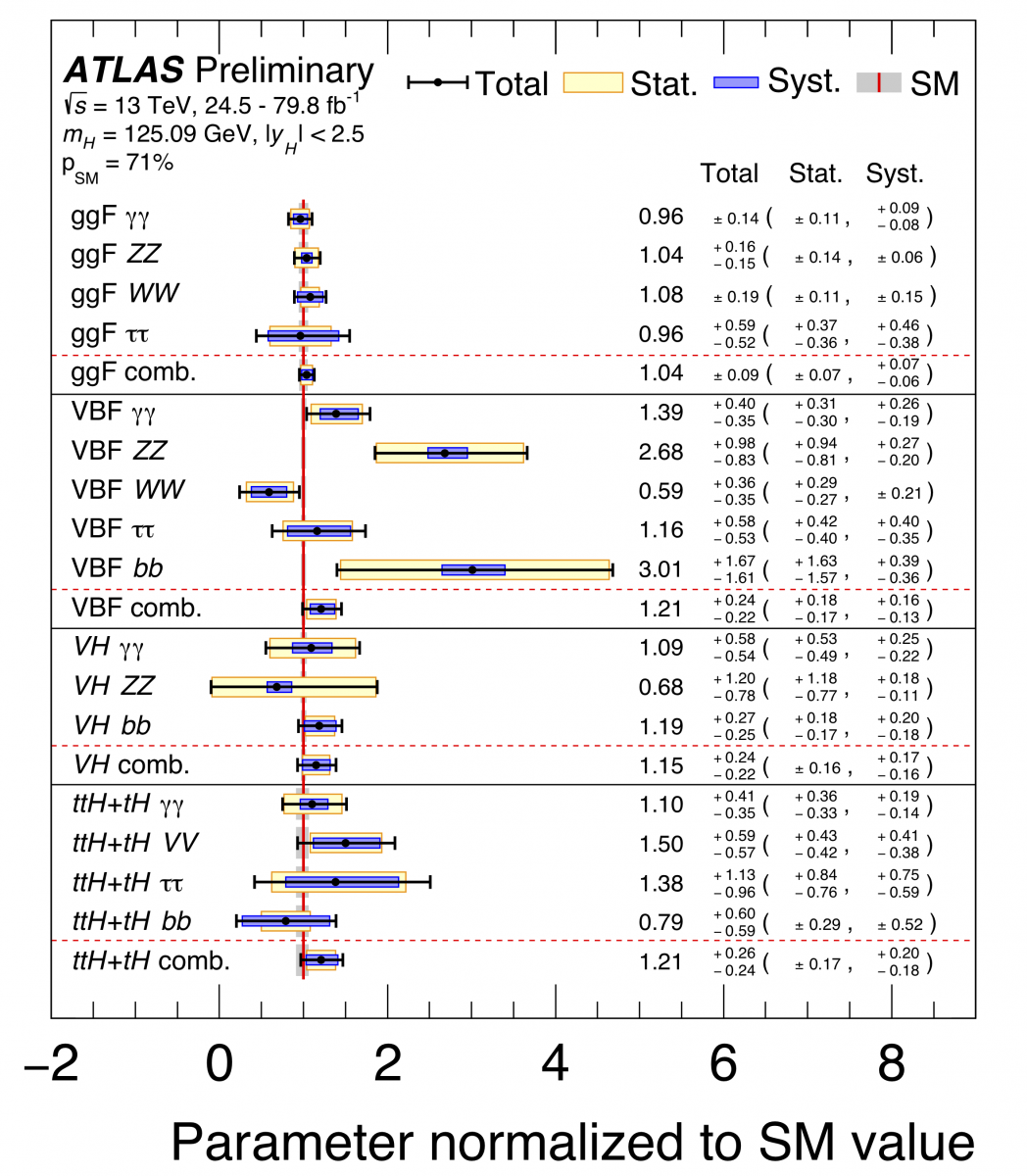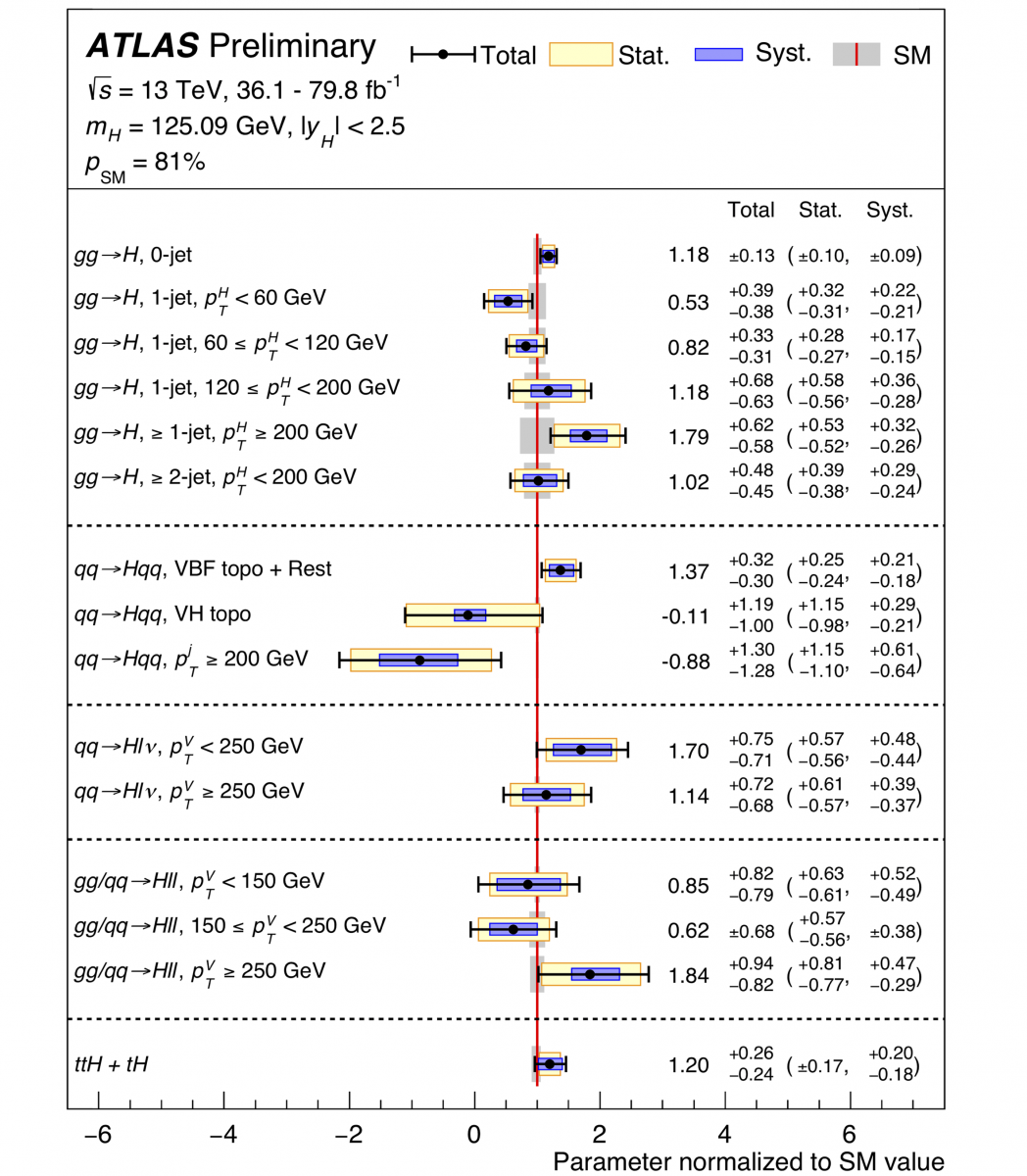Figure 1: Cross sections time branching fraction for the main Higgs production modes at the LHC (ggF, VBF, VH and ttH+tH) in each relevant decay mode (γγ, WW, ZZ, ττ, bb). All values are normalized to Standard Model predictions. In addition, the combined results for each production cross-section are also shown, assuming the Standard Model values for the branching ratios into each decay mode. (Image: ATLAS Collaboration/CERN)

The Higgs boson was discovered in 2012 by the ATLAS and CMS experiments, but its rich interaction properties (its coupling to other particles) remain a puzzle whose pieces the experiments on the Large Hadron Collider (LHC) are bringing together.

Fortunately, the LHC provides many windows into measuring Higgs boson couplings. There are four main ways to produce the Higgs boson: through the fusion of two gluon particles (gluon-fusion, or ggF); through the fusion of weak vector bosons (VBF); or in association with a W or Z boson (VH), or one or more top quarks (ttH+tH). There are also five main channels in which Higgs bosons can decay: into pairs of photons, W or Z bosons, tau leptons or b quarks. Each of these processes brings unique insights into the Higgs boson properties – and a separate piece in the puzzle of its true nature.

Thanks to the unprecedented amount of Higgs bosons produced at the LHC, all of the above production and decay modes have now been observed. In a new result presented by the ATLAS Collaboration, utilising data collected up to 2017, the measurements for each of these processes have reached the five standard deviation significance threshold, past which their existence is considered established.

The Higgs boson yields for most of the combinations of production and decay have been measured (see Figure 1) and have been found to agree with Standard Model predictions. The measurement of the cross sections for each production mode in proton–proton collisions at 13 TeV, assuming the decays occur as predicted by the Standard Model, are the most precise ones obtained to date.

### Thanks to the unprecedented amount of Higgs bosons produced at the LHC, all of the main Higgs boson production and decay modes have now been observed.Figure 2: Combined measurements of the cross section for the different production phase-space regions (STXS) considered in the analysis normalized by the Standard Model expectations. These regions are defined by different ranges of Higgs transverse momentum, number of associated jets, interval of vector boson transverse momentum in the VH production mechanism with the vector boson decaying leptonically. In this combination, the Higgs decay branching fractions are fixed to Standard Model values. (Image: ATLAS Collaboration/CERN)

As physicists have placed these new pieces, they’ve also begun to explore the Higgs boson puzzle in a new way. In the latest analyses, instead of counting Higgs bosons inclusively in the major production and decay modes, ATLAS physicists have measured Higgs boson topologies separately for smaller regions of phase-space: different ranges of Higgs boson transverse momentum, numbers of associated jets, and numbers and kinematic properties of associated weak bosons and top quarks. Using these smaller puzzle pieces, called "simplified template cross sections" (STXS), allows physicists to better separate the measurement process from the interpretation in terms of theoretical properties. Ultimately, it provides a finer-grained picture of Higgs boson couplings at the LHC and more stringent tests of the Standard Model.

Among the STXS regions considered in the analysis, some have already been measured with good precision at the LHC (see Figure 2), but no deviation from the Standard Model has been observed so far. These measurements allow physicists to further enhance the sensitivity on the coupling properties of the Higgs boson to the other elementary particles. Further, they have set constraints on new physics theories – such as the “two-Higgs doublet model”, which introduces additional Higgs bosons, and the hMSSM supersymmetric model – which are more stringent than those reported previously by ATLAS.

These measurements will continue to improve as more data from Run 2 and beyond are included, providing a yet-finer picture of the properties of the Higgs boson.